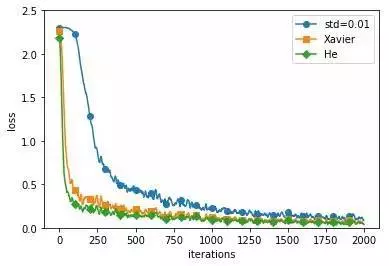2017-09-12 12:03:10 zhangjiaxuu 阅读数 748
• ###### 深度学习基础与TensorFlow实践教程

本课程主要介绍了深度学习的基础原理和TensorFlow系统基本使用方法。TensorFlow是目前机器学习、深度学习领域优秀的计算系统之一，本课程将结合实例介绍使用TensorFlow开发机器学习应用的详细方法和步骤，着重讲解了用于图像识别的卷积神经网络和用于自然语言处理的循环神经网络的理论知识及其TensorFlow实现方法，并结合实际场景和例子描述了深度学习技术的应用范围与效果。 所有案例均来自讲师团队工作中的亲身实践，所选案例均是深度学习的经典应用，非常具有代表性。

21447 人正在学习 去看看 AI100讲师

2：输入数据大小？

https://yq.aliyun.com/articles/118726?spm=5176.100239.blogcont122848.14.zwhUbk

import tensorflow as tf
import pandas as pd
import numpy as np
import os
import matplotlib
import matplotlib.pyplot as plt
import random
#matplotlib inline
import tensorflow as tf
import shutil
import tensorflow.contrib.learn as tflearn
import tensorflow.contrib.layers as tflayers
from tensorflow.contrib.learn.python.learn import learn_runner
import tensorflow.contrib.metrics as metrics
import tensorflow.contrib.rnn as rnn

random.seed(111)
rng=pd.date_range(start='2000',periods=209,freq='M')
"""pd.date_range 函数:生成日期范围
start：开始日期 periods:日期范围内日期的个数，periods*freq 等于日期范围的长度
freq：每多少天或其他明确时间频率的方式，默认为D，即1天 M为一个月
"""
ts=pd.Series(np.random.uniform(-10,10,size=len(rng)).cumsum())
ts.plot(c='b',title='Example Time Series')     #颜色蓝色
plt.show()

TS=np.array(ts)
num_periods=20              #观测值
f_horizon=1     #forecast horizon 预测一步

x_data=TS[:(len(TS)-len(TS)%num_periods)]          #len(TS)=209
x_batches=x_data.reshape(-1,20,1)

y_data=TS[1:(len(TS)-(len(TS)%num_periods))+f_horizon]
y_batches=y_data.reshape(-1,20,1)

print("x_data shape%s"%len(TS))
print("x_batchesis%s"%len(x_batches))             #10
print(x_batches.shape)                    # (10, 20, 1)
print("x_batches[0:2]is %s"%x_batches[0:2])

print("y_batches[0:1]is%s"%y_batches[0:1])
print(y_batches.shape)                      #(10, 20, 1)

##??不懂 测试数据是怎么取的 为什么
def test_data(series,forecast,num_periods):
test_x_setup=TS[-(num_periods+forecast):]
testX=test_x_setup[:num_periods].reshape(-1,20,1)
testY=TS[-(num_periods):].reshape(-1,20,1)
return testX,testY

X_test,Y_test=test_data(TS,f_horizon,num_periods)
print(X_test.shape)

print(X_test)

tf.reset_default_graph()              #清除默认图的堆栈，并设置全局图为默认图

num_periods=20
inputs=1
hidden=100
output=1

X=tf.placeholder(tf.float32,[None,num_periods,inputs])
y=tf.placeholder(tf.float32,[None,num_periods,output])

basic_cell=tf.contrib.rnn.BasicRNNCell(num_units=hidden,activation=tf.nn.relu)
rnn_output,states=tf.nn.dynamic_rnn(basic_cell,X,dtype=tf.float32)

learning_rate=0.001

"tf.layers.dense:全连接层 操作 outputs = activation(inputs.kernel + bias) "
stacked_rnn_output=tf.reshape(rnn_output,[-1,hidden])            #转为二维数据
stacked_outputs=tf.layers.dense(stacked_rnn_output,output)
outputs=tf.reshape(stacked_outputs,[-1,num_periods,output])              #?

loss=tf.reduce_sum(tf.square(outputs-y))
training_op=optimizer.minimize(loss)

init=tf.global_variables_initializer()

####################################
epochs=1000
with tf.Session() as sess:
init.run()
for ep in range(epochs):
sess.run(training_op,feed_dict={X:x_batches,y:y_batches})
if ep %100 ==0:
mse=loss.eval(feed_dict={X:x_batches,y:y_batches})
print(ep,"\TMSE:",mse)

y_pred=sess.run(outputs,feed_dict={X:X_test})
print(y_pred)

plt.title("Forecast vs Actual",fontsize=14)
plt.plot(pd.Series(np.ravel(Y_test)),"bo",markersize=10,label="Actual")
#plt.plot(pd.Series(np.ravel(Y_test)),"w*",markersize=10)
plt.plot(pd.Series(np.ravel(y_pred)),"r.",markersize=10,label="Forecast")
plt.legend(loc="upper left")
plt.xlabel("Time Periods")

plt.show()

2019-03-25 17:15:33 qq_37667364 阅读数 317
• ###### 深度学习基础与TensorFlow实践教程

本课程主要介绍了深度学习的基础原理和TensorFlow系统基本使用方法。TensorFlow是目前机器学习、深度学习领域优秀的计算系统之一，本课程将结合实例介绍使用TensorFlow开发机器学习应用的详细方法和步骤，着重讲解了用于图像识别的卷积神经网络和用于自然语言处理的循环神经网络的理论知识及其TensorFlow实现方法，并结合实际场景和例子描述了深度学习技术的应用范围与效果。 所有案例均来自讲师团队工作中的亲身实践，所选案例均是深度学习的经典应用，非常具有代表性。

21447 人正在学习 去看看 AI100讲师

	public static void main(String[] args) {
Sequential model = new Sequential();
//必须首先设置Input
model.setInput(new Input(x.length));
System.out.println("x_train:("+x.length+","+x.length+")");
//衰减可以使用默认的ExponentialLearningRateDecay.defaultDecay，默认lr初始0.2可以修改
//model.trainParallel(x, y, 400, 20, ExponentialLearningRateDecay.defaultDecay);
//衰减使用ExponentialLearningRateDecay.noDecay可以不进行lr的衰减，lr默认0.2可以修改
//model.trainParallel(x, y, 400, 20, ExponentialLearningRateDecay.noDecay);
ExponentialLearningRateDecay decay =
new ExponentialLearningRateDecay(2, 0.2, 0.8, false);
model.trainParallel(x, y, 400, 20, decay);
//以上参数迭代20个epoch后训练集acc=0.9860282
}

	/**
* 前向传播计算a和z这两个list
* 每个list保存了每一层的a或者z
* 只计算单个样本
* 每个样本的forwardPropagation可以并行，单个样本的forwardPropagation矩阵运算可以并行
* @return
*/
public ArrayList<double[]>[] forwardPropagation(double[] x) {
ArrayList<double[]>[] za = new ArrayList;
za = new ArrayList<>(layers.size());
za = new ArrayList<>(layers.size());
double[] input = x;
for(int i = 0; i < layers.size(); i++) {
double[][] weights = layers.get(i).getWeights();
double[] biases = layers.get(i).getBiases();
DoubleMatrix w = new DoubleMatrix(weights);
DoubleMatrix a = new DoubleMatrix(input);
DoubleMatrix b = new DoubleMatrix(biases);
// z = w*input + b
double[] zs = zMatrix.toArray();
//计算这一层的输出
ActivationFunction sigma = layers.get(i).getActivationFunction();
double[] as = sigma.functionValue(zs);
input = as;
}
return za;
}

/**
* 全连接层
* @author outsider
*
*/
public class Dense extends Layer{
//wij 指的时当前层第i个单元到上一层的j个单元连接的权重。
//所以weights的行数是本层的单元个数，列数是上一层单元的个数
private double[][] weights;
//偏置
private double[] biases;
//当前层的单元个数
private int units;
//激活函数
private ActivationFunction activationFunction;
public Dense(int units, ActivationFunction activationFunction) {
this.units = units;
this.activationFunction  = activationFunction;
}
/**
* 初始化权重参数
* @param lastUnits
*/
public void init(int lastUnits) {
this.biases = new double[units];
//权重随机初始化
this.weights = DoubleMatrix.randn(units, lastUnits).divi(10).toArray2();
DoubleMatrix doubleMatrix = new DoubleMatrix();
}

}


a的计算是把计算后的z送到对应层的激活函数中一个个计算a值。这里所有layer的a和z都要保存。保存z是因为中计算需要用到，保存a在计算会用到，和原理部分对应的。

	/**
*
* @param x 单个样本的x
* @param y 单个样本的y
* @return 每一层的梯度矩阵，注意是一个3维数组，每一个矩阵的尺寸不一样
*/
public Gradient backPropagation(double[] x, double[] y) {
//(1) 前向传播
ArrayList<double[]>[] za = forwardPropagation(x);
ArrayList<double[]> z = za;
ArrayList<double[]> a = za;
//(2) 反向传播计算 partial L / partial Z,这直接是偏置的梯度
double[][] LZ = new double[layers.size()][];
//计算最后一层的 partial L / partial z
LZ[LZ.length - 1] = layers.get(layers.size() - 1).getActivationFunction()
.partialLoss2PartialZ(null, a.get(a.size() - 1), y);
//计算其他层的 partial L / partial z
for(int i = LZ.length -2; i >=0; i--) {
Dense layer = layers.get(i);
//1.求激活函数的一阶导数
double[] sigmaP = layer.getActivationFunction().firstDerivativeValue(z.get(i));
//2.后一层的权重矩阵的转置乘以 partial L / partial z 向量
DoubleMatrix m1 = new DoubleMatrix(layers.get(i + 1).getWeights()).
transpose().mmul(new DoubleMatrix(LZ[i+1]));
// 1和2得到的向量对应元素相乘即为结果
LZ[i] = new DoubleMatrix(sigmaP).muli(m1).toArray();
}
//(3).计算梯度
//partial L / partial Z的 列向量乘以上一层的输入a行向量
double[] input = x;
for(int i = 0; i < gradients.length; i++) {
DoubleMatrix aVec = new DoubleMatrix(input);
DoubleMatrix lzVec = new DoubleMatrix(LZ[i]);
aVec = aVec.reshape(aVec.columns, aVec.rows);
input = a.get(i);
}
}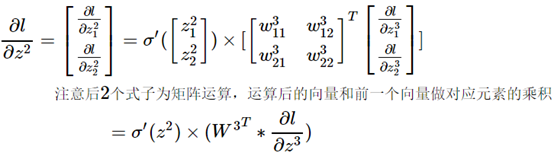/**
* 训练
* @param x
* @param y 每一个标签必须one-hot编码，所以是二维数组
* @param lr 学习率
* @param batchSize 每次梯度下降的更新参数用到的样本个数
* @param epochs 在整个样本集上做多少次的训练
* @param lrDecay 学习率指数衰减
*/
public void train(double[][] x, double[][] y,
int batchSize, int epochs, ExponentialLearningRateDecay lrDecay) {
//一个epoch中的各个batch可以并行，一个batch之间各单个样本之间可以并行
double lr = lrDecay.lr;
DoubleMatrix yM = new DoubleMatrix(y);
int[] yTrue = yM.rowArgmaxs();
this.classNum = layers.get(layers.size() - 1).getUnits();
//1.划分样本
int sampleNum = x.length;
//分多少批
int batchNum = sampleNum / batchSize;
System.out.println("batchNum:"+batchNum);
int left = sampleNum % batchSize;//样本余数
//保存样本的索引位置
List<Integer> sampleIndices = new ArrayList<>(x.length);
for(int i = 0; i < sampleNum; i++) {
}
// epoch循环
for(int i = 0; i < epochs; i++) {
//对学习率衰减
lr = lrDecay.decayLR(i);
System.out.println("epoch "+(i+1)+"/"+epochs+",lr="+lr);
//batch循环
for(int j = 0; j < batchNum; j++) {
int c = left *((j+1)/batchNum);
double[][] batchX = new double[batchSize + c][];
double[][] bathcY = new double[batchSize + c][];
System.out.println("epoch..."+(i+1)+"/"+epochs+",batch..."+(j+1)+"/"+batchNum+","+"batchSize="+batchX.length);
int offset = j * batchSize;
for(int k = j * batchSize; k < (j+1)*batchSize + c; k++) {
batchX[k-offset] = x[sampleIndices.get(k)];
bathcY[k-offset] = y[sampleIndices.get(k)];
}
//训练，一个一个样本计算梯度，这个过程可以同时进行。
//将每个样本的梯度累加
for(int m = 1; m < batchX.length; m++) {
for(int f = 0; f < weightsGradient.length; f++) {
}
}
//梯度除以N
for(int k = 0; k < layers.size(); k++) {
.divi(batchX.length).toArray();
divi(batchX.length).toArray2();
}
//更新参数
for(int f = 0; f < layers.size(); f++) {
Dense dense = layers.get(f);
double[][] oldW = dense.getWeights();
double[] oldBia = dense.getBiases();
// w = w - lr * gradient
double[][] newW = new DoubleMatrix(oldW).
double[] newBia = new DoubleMatrix(oldBia).
dense.setWeights(newW);
dense.setBiases(newBia);
}
//每20倍批次输出一次训练信息
/*if((j+1) % 20 == 0) {
int[] yPredicted = predictAndReturnClassIndex(x);
//训练集上的准确率
float acc = accuracy(yPredicted, yTrue);
System.out.println("\n"+(j+1)*batchSize + "/" + x.length+"........acc:"+acc+"\n");
}*/
}
double[][] yPre = predictParallel(x);
int[] yPredicted = new DoubleMatrix(yPre).rowArgmaxs();
double loss = loss(yPre, y);
float acc = accuracy(yPredicted, yTrue);
String print = "epoch " +(i+1)+ " done"+"........acc:"+acc+",total loss:"+loss;
System.out.println(print);
//打乱数据位置
//acc会有一些不稳定，估计也和这个操作有关系。
//测试后发现，shuffle有利用梯度下降
Collections.shuffle(sampleIndices);
}
}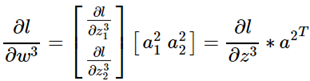1.遇到的问题：nn参数初始化

-0.25到0.25之间，关于参数初始化也有很大的学问，好的

2.遇到的问题
//之前不work主要有两个原因：
//（1）梯度最后一步计算的矩阵化公式有错
//（2）损失函数没有除以N（我不知道为什么这个影响这么大，这直接导致了效果完全不行）
//目前存在的问题
//（1）效率不够高
//（2）收敛不够快，前面设置的参数情况下，epoch 2才达到0.82左右的acc
//3.23更新：
//使用了学习率指数衰减，效果好多了
//参数建议，如果epochs比较小，那么lr也要小一点
3.关于并行

3.线程级别的计算基于单个样本还是多个样本？

4.并行对比
//train_parallel:4m22s one epoch
//train:7m07s
//train_parallel 比 train快1.63倍

5.关于并行训练的实现

6.使用方法

model = new Sequential()
.....
model.train()

2019-03-25 16:30:54 qq_37667364 阅读数 391
• ###### 深度学习基础与TensorFlow实践教程

本课程主要介绍了深度学习的基础原理和TensorFlow系统基本使用方法。TensorFlow是目前机器学习、深度学习领域优秀的计算系统之一，本课程将结合实例介绍使用TensorFlow开发机器学习应用的详细方法和步骤，着重讲解了用于图像识别的卷积神经网络和用于自然语言处理的循环神经网络的理论知识及其TensorFlow实现方法，并结合实际场景和例子描述了深度学习技术的应用范围与效果。 所有案例均来自讲师团队工作中的亲身实践，所选案例均是深度学习的经典应用，非常具有代表性。

21447 人正在学习 去看看 AI100讲师

## 1.激活函数

（1）Sigmoid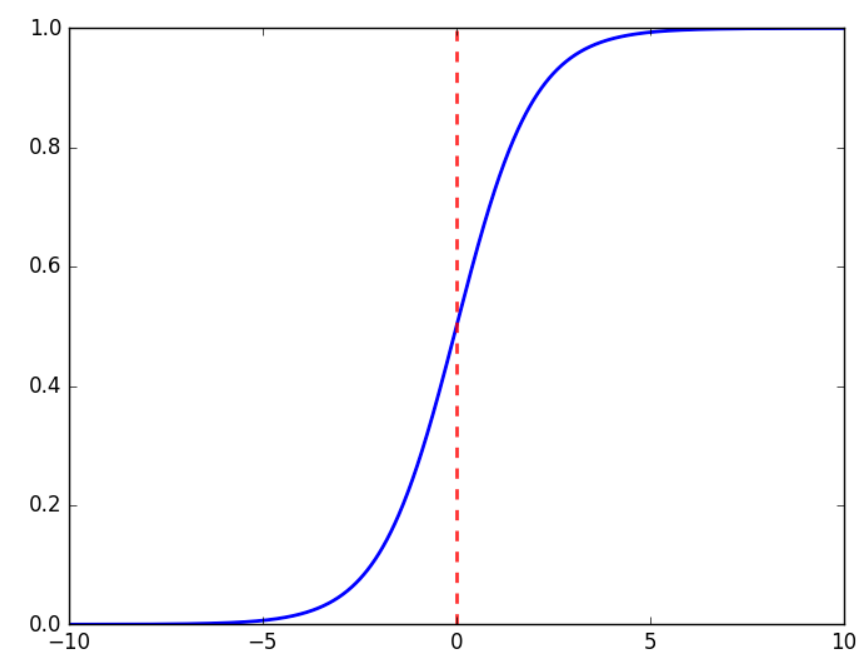Sigmoid将所有输入压缩到0到1之间，这样造成的问题是，当随着神经网络的层次加深时，特征的变化由于sigmoid而慢慢衰减。这样会造成靠近输入层的梯度很小。

（2）ReLU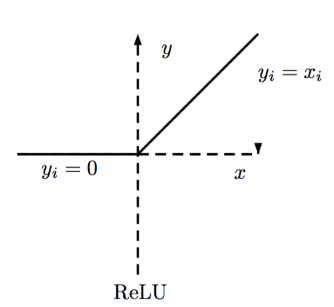ReLU是现在DL流行使用的激活函数，有一些变种。

ReLU实际上是一种线性的函数，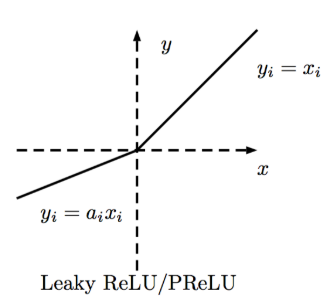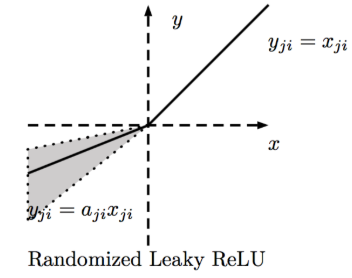（3）MaxOut

MaxOut可以自适应的训练出激活函数。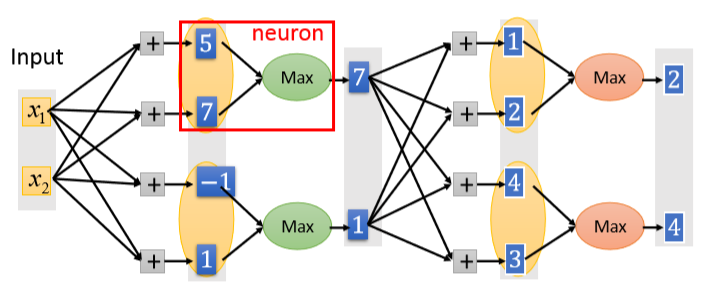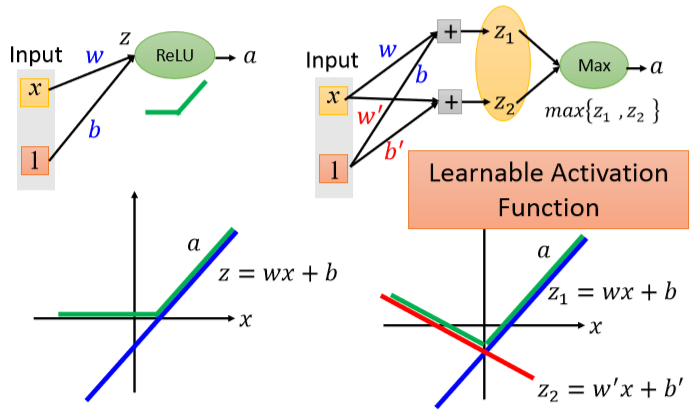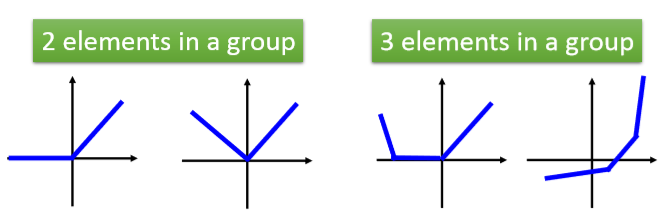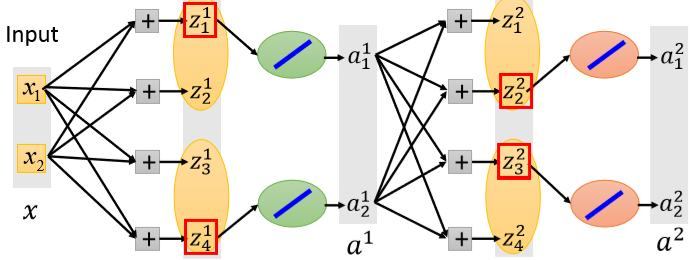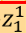被选中为最大，那么其对应的激活函数就是线性的，会更新对应的权重，输入不同的样本，可能选中不同的z，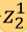也可能会被选中更新参数。

## 2.batch size 和 epochs

batch size的来源：将样本分批，每一批样本用来优化损失函数，这其实就是批量梯度下降，分批最好随机。。比如样本有10000个，batch size = 100，那么分批为100批，优化时需要做100次参数更新。

epochs:将样本分批后，一次epoch就是用完所有批次的样本用来梯度下降。例如：样本10000，batch size = 100，epochs = 10，每一个epoch参数更新100次，共10个epoch。

1. batch size = 1，每一个epoch梯度下降10000次，更新10000次参数
2. batch  size = 10，每一个epoch梯度下降1000次，更新1000次参数

## 3.过拟合处理（正则和dropout）

（1）正则

（2）dropout（提升模型泛化能力）

1）什么是dropout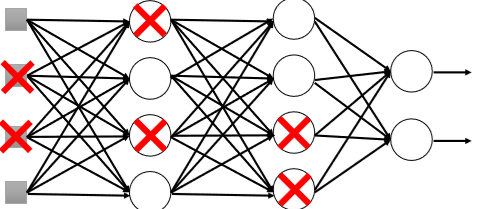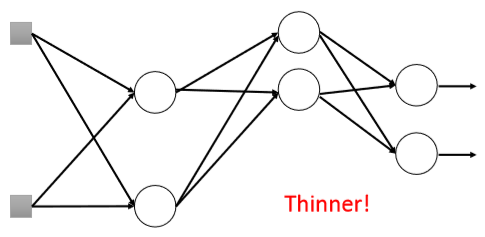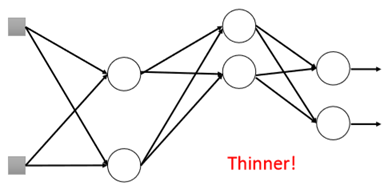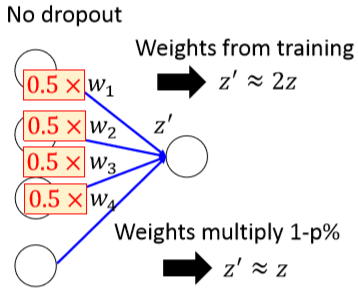2）为什么dropout有效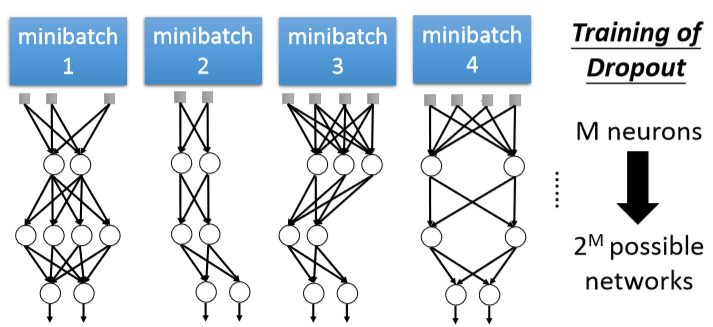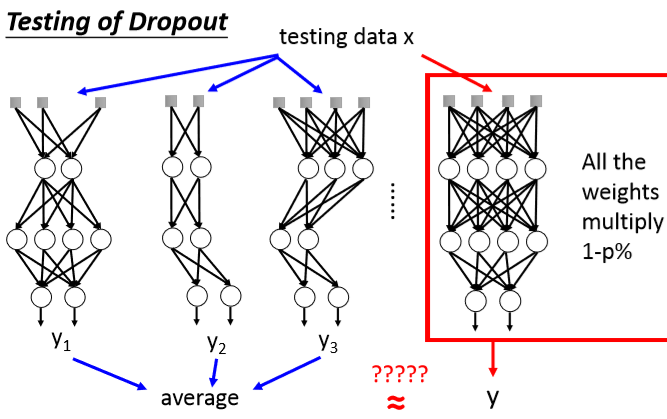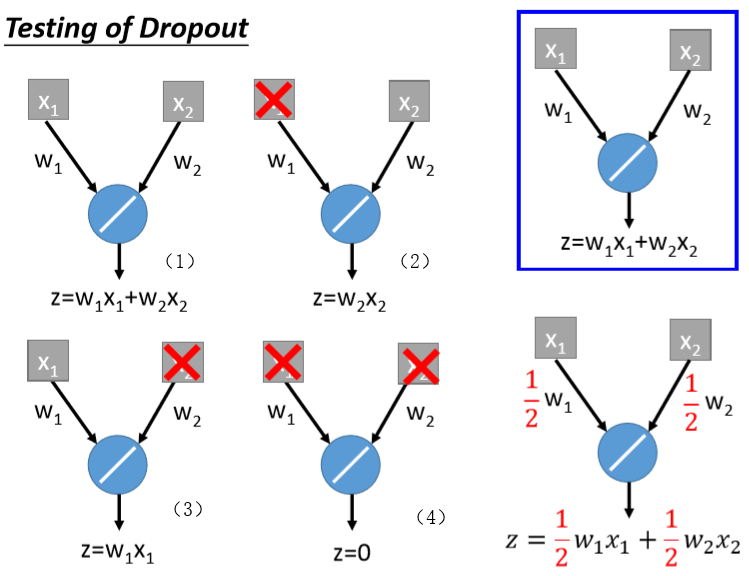（1）（2）（3）（4）出现的可能都是1/4。例如（1）x1不被丢掉的概率是0.5，x2不被丢掉的概率是0.5，所以情况（1）出现的可能是0.5*0.5=0.25=1/4。总和计算4中情况的z结果，就是算一个z的期望，

0.25*z(1)+ 0.25*z(2)+ 0.25*z(3)+ 0.25*z(4) = 1/2w1x1+1/2w2x2

## 3.训练DL模型的建议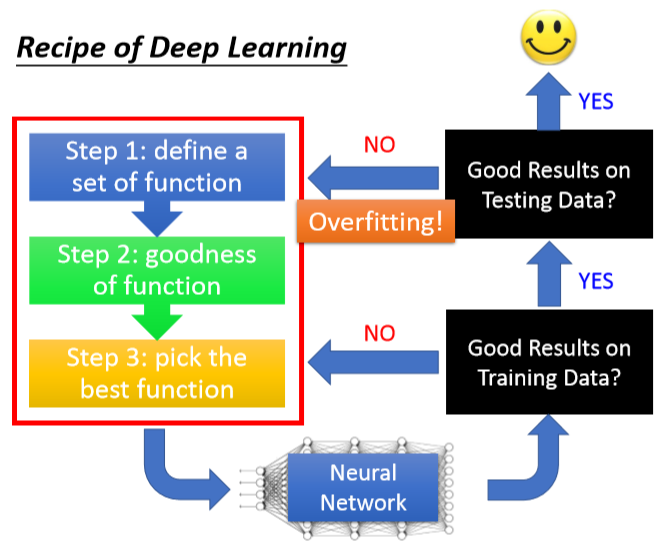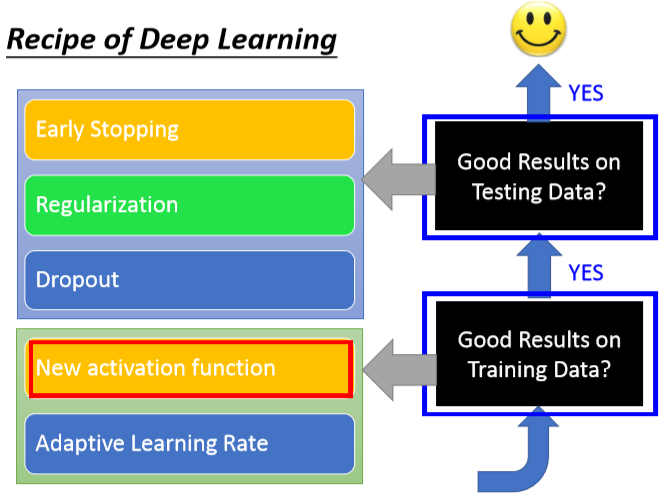Keras注意事项：

1. 做分类时，label必须要one-hot编码
2. 首先关注在训练集上的效果，如果训练集上的效果都不好，那么一定有问题，如果训练集上拟合得比较好，但测试集不太好，这时可以考虑是不是过拟合了
3. 损失函数的选择
4. 激活函数的选择（见激活函数小节）

2019-03-24 21:53:55 qq_40522828 阅读数 90
• ###### 深度学习基础与TensorFlow实践教程

本课程主要介绍了深度学习的基础原理和TensorFlow系统基本使用方法。TensorFlow是目前机器学习、深度学习领域优秀的计算系统之一，本课程将结合实例介绍使用TensorFlow开发机器学习应用的详细方法和步骤，着重讲解了用于图像识别的卷积神经网络和用于自然语言处理的循环神经网络的理论知识及其TensorFlow实现方法，并结合实际场景和例子描述了深度学习技术的应用范围与效果。 所有案例均来自讲师团队工作中的亲身实践，所选案例均是深度学习的经典应用，非常具有代表性。

21447 人正在学习 去看看 AI100讲师

## 深度学习的应用

NP（自然语言）处理

1.交叉验证

2.剪枝

3.正则化

L2（如果不在意对于特征分析）

S函数：

### 使用jupyter notebook来一步一步执行，观察结果

#### 用深度学习来做线性回归问题

In:	import numpy as np
import tensorflow as tf
import matplotlib.pyplot as plt

In:	# 随机生成一千个点，围绕在y=0.1x+0.3的直线范围内
num_points = 1000
vectors_set = []
for i in range(num_points):
# 生成一个均值为0.0，方差为0.55的高斯分布
x1 = np.random.normal(0.0, 0.55)
# 声明一个y=0.1x+0.3的函数，增加一些抖动(wx+b)
y1 = x1*0.1 + 0.3 + np.random.normal(0.0, 0.03)
# 放入vectors_set中
vectors_set.append([x1,y1])

In:	# 生成一些样本
x_data = [v for v in vectors_set]
y_data = [v for v in vectors_set]
plt.scatter(x_data, y_data)
plt.show()

In:	# 模拟训练
# 生成一个一维的w矩阵，取值范围在[-1,1]之间
W = tf.Variable(tf.random_uniform(, -1, 1), name='W')
print(W)

In:	# 生成一个一维的矩阵b，初始值为0
b = tf.Variable(tf.zeros(), name='b')

In:	# 经过计算得出预估值y
y = W * x_data + b

In:	# 以预估值y和实际值y_data之间的协方差作为损失
loss = tf.reduce_mean(tf.square(y-y_data), name='loss')
# 使用梯度下降法来优化参数
# 训练的过程就是最小化这个误差值
train = op.minimize(loss, name='train')
# 建立会话
sess = tf.Session()
# 全局变量的初始化
init = tf.global_variables_initializer()
# 初始化训练变量
sess.run(init)
# 查看初始化的W和b的值
print('W=', sess.run(W), 'b=', sess.run(b), 'loss=', sess.run(loss))

In:	# 进行训练，训练30次
for step in range(30):
sess.run(train)
# 输出训练完的W和b的值，看一下能否接近0.1和0.3
print('W=', sess.run(W), 'b=', sess.run(b), 'loss=', sess.run(loss))

In:	# 完成线性回归
plt.scatter(x_data, y_data)
plt.plot(x_data, sess.run(W)*x_data + sess.run(b), c='r')
plt.show()


#### 深度学习实现逻辑回归

In:	import numpy as np
import tensorflow as tf
import matplotlib.pyplot as plt
import tensorflow.examples.tutorials.mnist.input_dataput_data as input_data

In:	mnist = input_data.read_data_sets('data/', one_hot=True)
print(mnist)

In:	# 下载下来的数据集被分为三个子集
# 5.5W行的训练数据集（mnist.train）
# 五千行的验证数据集(mnist.validation)
# 一万行的测试数据集(mnist.test)
# 因为每张图片为28X28的黑白照片，所以每行为784维的向量
trainimg = mnist.train.images
trainlabel = mnist.train.labels
testimg = mnist.test.images
testlabel = mnist.test.labels
print(mnist.validation.labels.shape)

In:	print(trainimg.shape)
print(trainlabel.shape)
print(testimg.shape)
print(testlabel.shape)
print(trainlabel)

In:	x = tf.placeholder('float', [None, 784]) # 784是维度，None表示无限多
y = tf.placeholder('float', [None, 10])
W = tf.Variable(tf.zeros([784, 10])) # 每个数字是784个像素点的，所以w和x相乘的话也要有784个，b=10表示这个是十分类的
b = tf.Variable(tf.zeros())
# 回归模型
actv = tf.nn.softmax(tf.matmul(x, W) + b) # 将逻辑回归升级为softmax，x*W+b相当于得到y，每次x*W+b之后都要经过激活函数
# cost function 总和 均值
cost = tf.reduce_mean(-tf.reduce_sum(y*tf.log(actv), reduction_indices=1))
# 优化
learning_rate = 0.1

In:	pred = tf.equal(tf.argmax(actv, 1), tf.argmax(y, 1)) # 看一下预测值与真实值是否一样
# 正确率
accr = tf.reduce_mean(tf.cast(pred, 'float')) # tf.cast将True变为1，False变为0
# 初始化
init = tf.global_variables_initializer()

In:	sess = tf.InteractiveSession()
arr = np.array([[31, 23, 4, 24, 27, 34],
[18, 3, 25, 0, 6, 35],
[28, 14, 33, 22, 20, 8],
[13, 30, 21, 19, 7, 9],
[16, 1, 26, 32, 2, 29],
[17, 12, 5, 11, 10, 15]])
tf.rank(arr).eval() # 返回矩阵的维度，在tensorflow中打印要加.eval()
tf.shape(arr).eval()
tf.argmax(arr,0).eval() # 返回最大值的索引，0表示axis=0，按列求解

In:	# 每多少次迭代显示一次损失
training_epochs = 50
# 批尺寸
batch_size = 100
# 训练迭代次数
display_step = 5
# session
sess = tf.Session()
sess.run(init)

for epoch in range(training_epochs):
avg_cost = 0
# 55000/100
num_batch = int(mnist.train.num_examples/batch_size)
for i in range(num_batch):
# 获取数据集  next_batch:获取下一批数据
batch_xs, batch_ys = mnist.train.next_batch(batch_size)
# 模型训练
sess.run(optm, feed_dict={x:batch_xs, y:batch_ys})
feeds = {x:batch_xs, y:batch_ys}
avg_cost += sess.run(cost, feed_dict=feeds) / num_batch
# 满足5次的一个迭代
if epoch % display_step == 0:
feed_train = {x:batch_xs, y:batch_ys}
feed_test = {x:mnist.test.images, y:mnist.test.labels}
train_acc = sess.run(accr, feed_dict=feed_train)
test_acc = sess.run(accr, feed_dict=feed_test)
print('Epoch:%03d/%03d cost: %.9f train_acc:%.3f test_acc: %.3f' % (epoch, training_epochs, avg_cost, train_acc, test_acc))
print('Done')


#### 深度学习手写数字

In:	import numpy as np
import matplotlib.pyplot as plt
import tensorflow as tf
import tensorflow.examples.tutorials.mnist.input_data as input_data

In:	mnist = input_data('data1/', one_hot=True) # 从网上下载数据，放在data1中
print('type of mnist is %s' % type(mnist))
print('number of train data is %d' % (mnist.train.num_examples)) # input_data里自带统计数据集数量的函数
print('number of test data is %d' % (mnist.test.num_examples))

In:	# 显示mnist数据集的内容
trainimg = mnist.train.images
trainlabel = mnist.train.labels
testimg = mnist.test.images
testlabel = mnist.test.labels

print('type of mnist.train.images is %s' % type(trainimg))
print('type of mnist.train.labels is %s' % type(trainlabel))
print('type of mnist.test.images is %s' % type(testimg))
print('type of mnist.test.labels is %s' % type(testlabel))

print('type of mnist.train.images is %s' % (trainimg.shape,)) # 将28*28像素的图片 变成784*1   将28行变成1行
print('type of mnist.train.labels is %s' % (trainlabel.shape,))
print('type of mnist.test.images is %s' % (testimg.shape,))
print('type of mnist.test.labels is %s' % (testlabel.shape,))
# 要加，号 不然会报错

In:	# 操作数据集
# 数据图像化
nsample = 5
randix = np.random.randint(trainimg.shape, size=nsample) # 在55000中随机选出5个数
for i in randix:
curr_img = np.reshape(trainimg[i, :], (28,28)) # 28*28的矩阵
curr_label = np.argmax(trainlabel[i, :]) # label
plt.matshow(curr_img, cmap=plt.get_cmap('gray')) # cmap=plt.get_cmap('gray')指定画出的图是灰色的
plt.title(str(i) + 'th Training Data,Label is ' + str(curr_label))
plt.show()

In:	# batch学习
batch_size = 100
batch_xs, batch_ys = mnist.train.next_batch(batch_size)
print(type(batch_xs))
print(type(batch_ys))

print(batch_xs.shape)
print(batch_ys.shape) # 有十个类属于这一类的数字为1，其余为0


#### SimpleNeuralNetwork

In:	import numpy as np
import tensorflow as tf
import matplotlib.pyplot as plt
import tensorflow.examples.tutorials.mnist.input_data as input_data

In:	mnist = input_data.read_data_sets('data/', one_hot=True)

In:	# network topologies
n_hidden_1 = 256 # 第一个隐藏层的神经元数
n_hidden_2 = 128 # 第二个隐藏层的神经元数
n_input = 784 # 输入的像素
n_classes = 10 # 输出的label
# input and output
x = tf.placeholder('float', [None, n_input]) # 入口数据
y = td.placeholder('float', [None, n_classes])

# 神经网络
stddev=0.1
weights = {
'w1':tf.Variable(tf.random_normal([n_input, n_hidden_1], stddev=stddev)),
'w2':tf.Variable(tf.random_normal([n_hidden_1, n_hidden_2], stddev=stddev)),
'out':tf.Variable(tf.random_normal([n_hidden_2, n_classes], stddev=stddev))
}
biases = {
'b1':tf.Variable(tf.random_normal([n_hidden_1])),
'b2':tf.Variable(tf.random_normal([n_hidden_2])),
'out':tf.Variable(tf.random_normal([n_classes]))
}
print('network hard')
print(weights['w1'])

In:	# s激活函数，进行数据的操作(BP算法)
def multilayer_perceotron(_X, _weights, _biases):
layer_1 = tf.nn.sigmoid(tf.add(tf.matmul(_X, _weights['w1']), _biases['b1'])) # 每次wx+b之后经过sigmoid函数激活
return (tf.matmul(layer_2, _weights['out']) + _biases['out']) # 返回的是10个输出

In:	pred = multilayer_perceotron(x, weights, biases)
# 损失函数 softmax_cross_entropy_with_logits中0.x版本和1.x版本的不同的是1.x版本要加上logits和labels
cost = tf.reduce_mean(tf.nn.softmax_cross_entropy_with_logits(logits=pred, labels=y))
# 如果相等返回true，不等返回false
corr = tf.equal(tf.argmax(pred, 1), tf.argmax(y, 1)) # argmax是返回每行最大的数的列名，1的意思是axis=1
# 把true转换为1，false为0，再相加，计算总的（正确率）
accr = tf.reduce_mean(tf.cast(corr, 'float'))
# 初始化
init = tf.global_variables_initializer()

In:	# 执行模型的训练
training_epochs = 20 # 迭代的次数
batch_size = 100 # 每次处理的图片数
display_step = 4 # 每四次打印一次
# launch the graph
sess = tf.Session()
sess.run(init)
# optimize
for epoch in range(training_epochs):
avg_cost = 0. # 平均误差
total_batch = int(mnist.train.num_examples / batch_size)
# iteration 训练集循环次数的确定
for i in range(total_batch):
# 取出x和y的值
batch_xs, batch_ys = mnist.train.next_batch(batch_size)
feeds = {x:batch_xs, y:batch_ys}
sess.run(optm, feed_dict=feeds)
avg_cost += sess.run(cost, feed_dict=feeds)
avg_cost = avg_cost / total_batch # 更新平均误差
# display 显示误差率和训练集正确率以及测试集的正确率
if (epoch+1) % display_step == 0:
print('Epoch:%03d/%03d cost:%.9f' % (epoch, training_epochs, avg_cost))
feeds = {x:batch_xs, y:batch_ys}
train_acc = sess.run(accr, feed_dict=feeds)
print('TRAIN ACCURACY:%.3f' % (train_acc))
feeds = {x:mnist.test.images, y:mnist.test.labels}
test_acc = sess.run(accr, feed_dict=feeds)
print('TEST ACCURACY:%.3f' % (test_acc))
print('optimization finished')
# 最后的损失函数下降得不是很多，正确率也不高，需要加大迭代次数


2019-12-08 16:13:29 weixin_43838785 阅读数 11
• ###### 深度学习基础与TensorFlow实践教程

本课程主要介绍了深度学习的基础原理和TensorFlow系统基本使用方法。TensorFlow是目前机器学习、深度学习领域优秀的计算系统之一，本课程将结合实例介绍使用TensorFlow开发机器学习应用的详细方法和步骤，着重讲解了用于图像识别的卷积神经网络和用于自然语言处理的循环神经网络的理论知识及其TensorFlow实现方法，并结合实际场景和例子描述了深度学习技术的应用范围与效果。 所有案例均来自讲师团队工作中的亲身实践，所选案例均是深度学习的经典应用，非常具有代表性。

21447 人正在学习 去看看 AI100讲师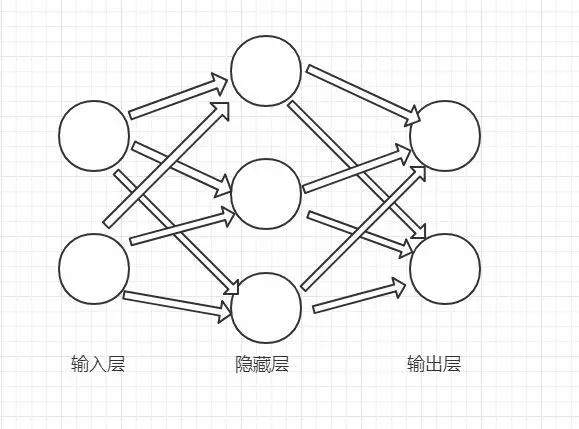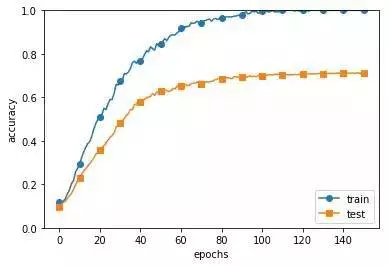Dropout优化

Momentum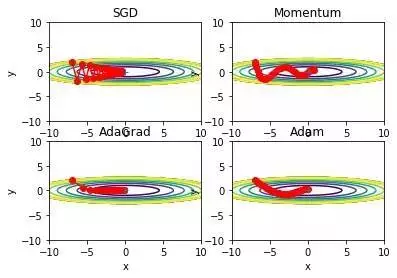Xavier初始值：适用于激活函数是sigmoid函数

He初始值：适用于激活函数是ReLU函数